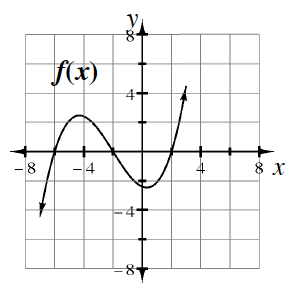### Home > INT3 > Chapter 8 > Lesson 8.3.1 > Problem8-102

8-102.

Each graph below represents a polynomial function. For each function, state its minimum degree. Then state the number of real and complex roots it has.

Example: The graph of $y = f\left(x\right)$ at right has degree $3$. It has three real roots and no complex roots.

1.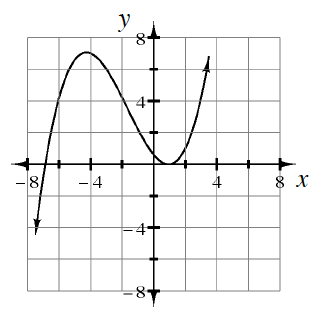Degree $3$
There are two distinct real roots, one of which is repeated.

1.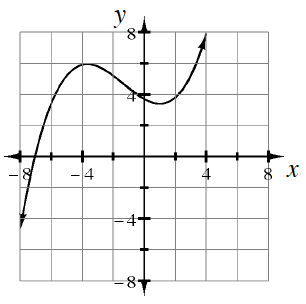1.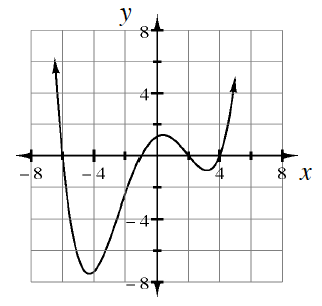1.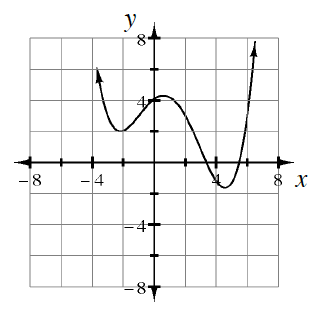Degree $4$
There are two real roots and two complex roots.